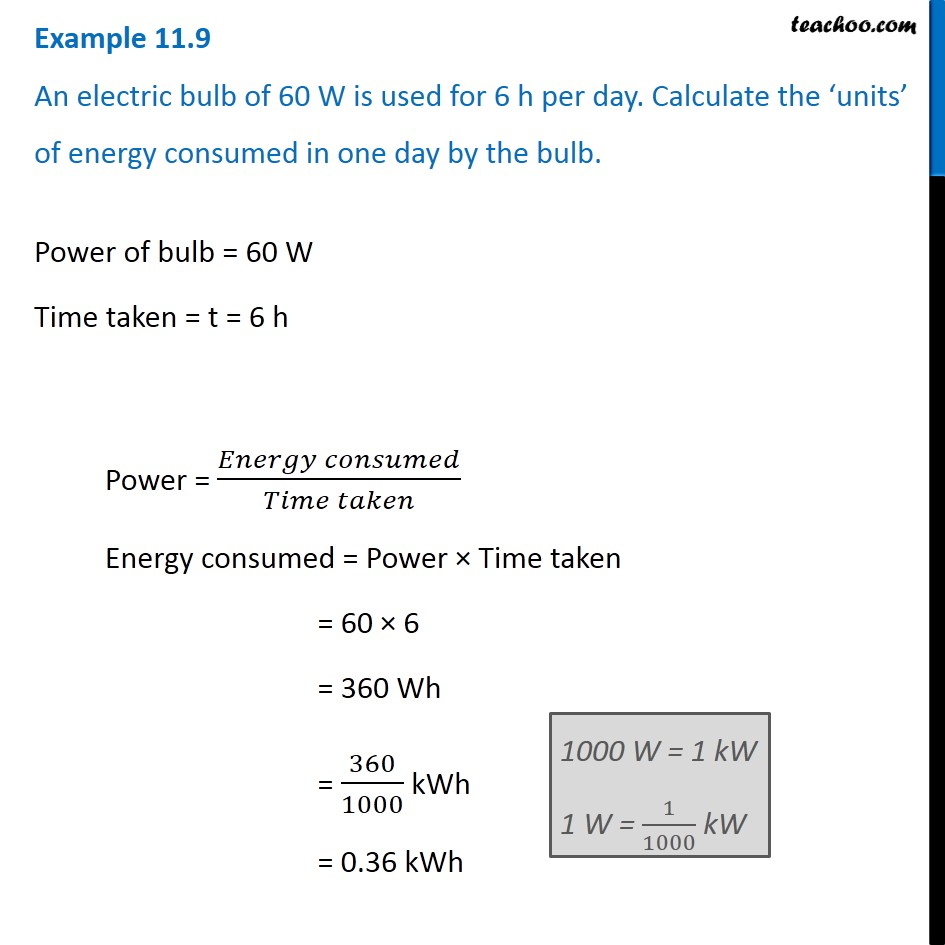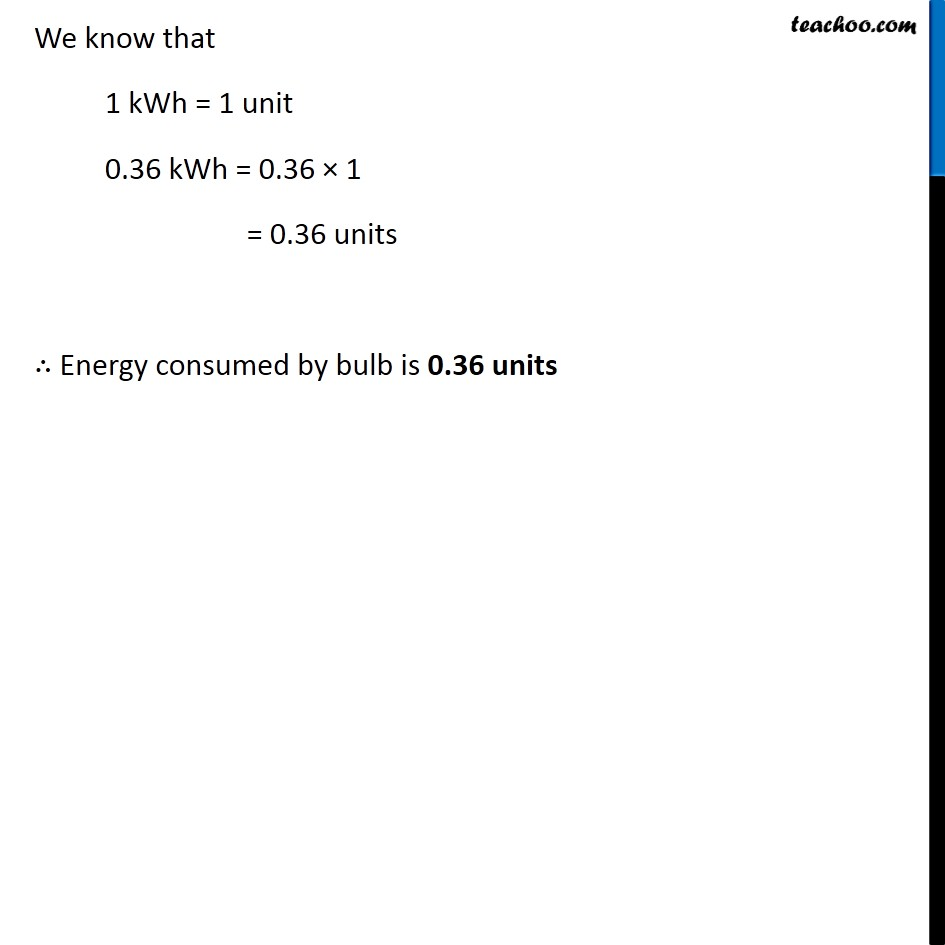Examples from NCERT Book

Class 9
Chapter 11 Class 9 - Work and EnergyLearn in your speed, with individual attention - Teachoo Maths 1-on-1 Class

### Transcript

Example 11.9 An electric bulb of 60 W is used for 6 h per day. Calculate the ‘units’ of energy consumed in one day by the bulb. Power of bulb = 60 W Time taken = t = 6 h Power = (𝐸𝑛𝑒𝑟𝑔𝑦 𝑐𝑜𝑛𝑠𝑢𝑚𝑒𝑑)/(𝑇𝑖𝑚𝑒 𝑡𝑎𝑘𝑒𝑛) Energy consumed = Power × Time taken = 60 × 6 = 360 Wh = 360/1000 kWh = 0.36 kWh 1000 W = 1 kW 1 W = 1/1000 kW We know that 1 kWh = 1 unit 0.36 kWh = 0.36 × 1 = 0.36 units ∴ Energy consumed by bulb is 0.36 units